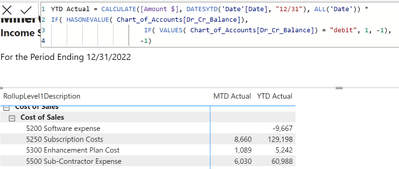cancel
Showing results for
Did you mean:New Member

## Hasonevalue not working

I have a field name called Dr_Cr_Balance, which only has one value in the Chart of Accounts table. In the following expression I am testing to see if Hasonevalue = "debit" or "credit". If "debit, I want to multiple the TOTALYTD by 1, and if the value in the Chart of Accounts is "credit" then multiply it by -1.  The expression works as long as there is a number in the MTD Actual column. If this is blank, then the YTD Actual has the wrong sign. Anyone have any ideas on how to fix this?5 REPLIES 5New Member

Can anyone help with the formula and the error message in my last message?Super User

@pfernandez , It is better to have a measure like

Calculate(

calculate(1* [Amount \$], filter(ChartofAccount, ChartofAccount[Dr_cr_flag]= "Dr")  +

calculate(-1* [Amount \$], filter(ChartofAccount, ChartofAccount[Dr_cr_flag]= "Cr"),  Dateytd(Date[Date]) )

I do not think you all date , make sure date table is marked as date table and used in visual if needed

Why Time Intelligence Fails - Powerbi 5 Savior Steps for TI :https://youtu.be/OBf0rjpp5Hw
https://amitchandak.medium.com/power-bi-5-key-points-to-make-time-intelligence-successful-bd52912a5b...New Member

Hi Amitchandak, I tried this and I probably have a mistake somewhere in the formula. Can you take a look and help me fix this?New Member

It looks like i was missing a comma in the second CALCULATE function. I added the comma and now I have this:

YTD Actual = CALCULATE(1 * [Amount \$], FILTER(Chart_of_Accounts, Chart_of_Accounts[Dr_Cr_Balance] - "debit") + CALCULATE (-1 * [Amount \$], FILTER(Chart_of_Accounts, Chart_of_Accounts[Dr_Cr_Balance] = "credit", DATESYTD('Date'[Date], "12/31"), ALL('Date'))))
I am not getting a message saying "too many arguments were passed to the FILTER function. The maximum argument count for the function is 2."New Member

I am still looking for a solution. Does anyone know how to fix the above DAX statement?